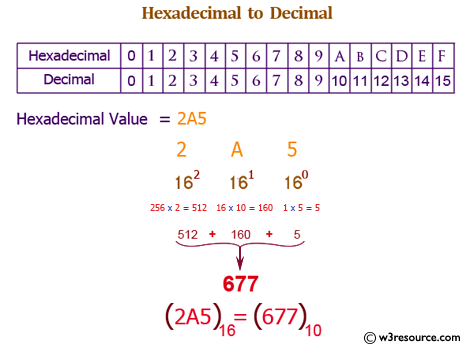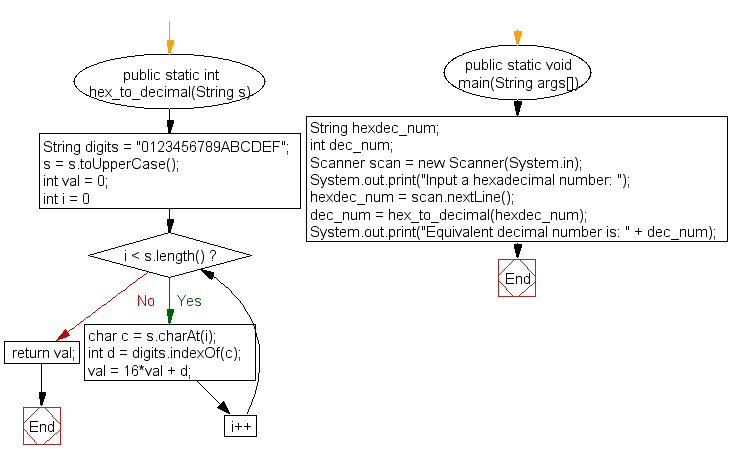﻿ Java exercises: Convert a hexadecimal to a decimal number - w3resource# Java Exercises: Convert a hexadecimal to a decimal number

## Java Basic: Exercise-28 with Solution

Write a Java program to convert a hexadecimal to a decimal number.

Hexadecimal number: This is a positional numeral system with a radix, or base, of 16. Hexadecimal uses sixteen distinct symbols, most often the symbols 0-9 to represent values zero to nine, and A, B, C, D, E, F (or alternatively a, b, c, d, e, f) to represent values ten to fifteen.

Decimal number: The decimal numeral system is the standard system for denoting integer and non-integer numbers. It is also called base-ten positional numeral system.

Test Data:

Pictorial Presentation: Hexadecimal to Decimal numberSample Solution:

Java Code:

``````import java.util.Scanner;

public class Exercise28 {
public static int hex_to_decimal(String s)
{
String digits = "0123456789ABCDEF";
s = s.toUpperCase();
int val = 0;
for (int i = 0; i < s.length(); i++)
{
char c = s.charAt(i);
int d = digits.indexOf(c);
val = 16*val + d;
}
return val;
}
public static void main(String args[])
{
String hexdec_num;
int dec_num;
Scanner scan = new Scanner(System.in);

hexdec_num = scan.nextLine();

dec_num = hex_to_decimal(hexdec_num);

System.out.print("Equivalent decimal number is: " + dec_num+"\n");
}
}
```
```

Sample Output:

```Input a hexadecimal number: 25
Equivalent decimal number is: 37
```

Flowchart:Java Code Editor:

What is the difficulty level of this exercise?

Test your Programming skills with w3resource's quiz.

﻿

## Java: Tips of the Day

countOccurrences

Counts the occurrences of a value in an array.

Use Arrays.stream().filter().count() to count total number of values that equals the specified value.

```public static long countOccurrences(int[] numbers, int value) {
return Arrays.stream(numbers)
.filter(number -> number == value)
.count();
}
```

Ref: https://bit.ly/3kCAgLb